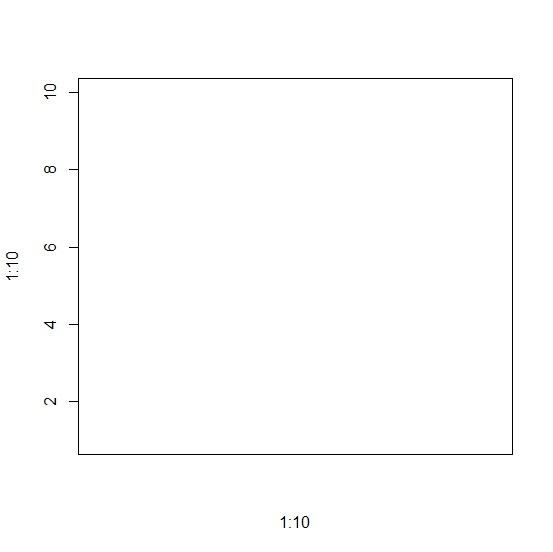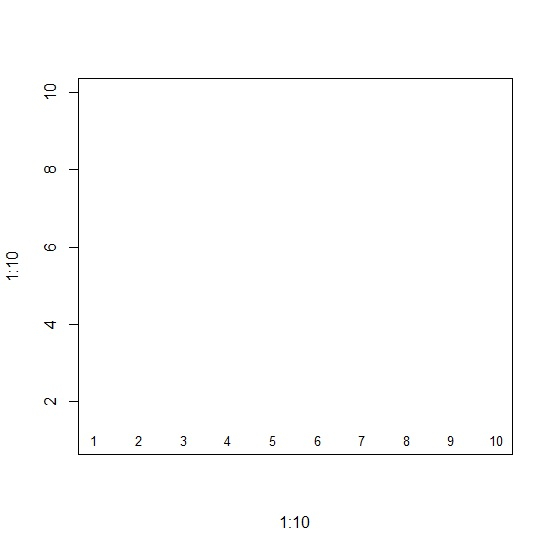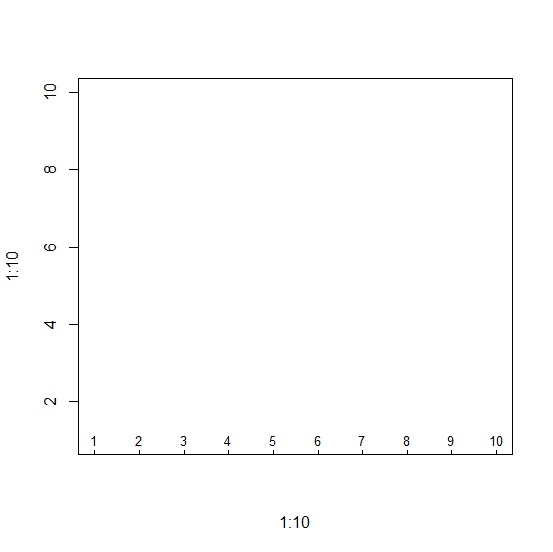# How to display X-axis labels inside the plot in base R?

To display X-axis labels inside the plot in base R, we can follow the below steps −

• First of all, create a plot without X-axis labels and ticks.

• Then, display the labels inside the plot.

• After that, display the ticks inside the plot.

## Create the plot

Using plot function, create a plot without X-axis labels and ticks −

Live Demo

plot(1:10,1:10,xaxt="n",type="n")

## Output## Display the axis labels inside the plot

Using text function to display the X-axis labels inside the plot −

Live Demo

plot(1:10,1:10,xaxt="n",type="n")
text(1:10,1,1:10,cex=0.8)

## Output## Display the axis ticks inside the plot

Using axis function to display the X-axis ticks inside the plot −

Live Demo

plot(1:10,1:10,xaxt="n",type="n")
text(1:10,1,1:10,cex=0.8)
axis(1,at=c(1:10),NA,cex.axis=0.8,tck=0.01)

## Output